Published: 15 May 2019

# Stochastic P-bifurcation in a tri-stable Van der Pol system with fractional derivative under Gaussian white noise

Yajie Li1
Zhiqiang Wu2
1, 2Department of Mechanics, School of Mechanical Engineering, Tianjin University, Tianjin, 300072, China
1, 2Tianjin Key Laboratory of Nonlinear Dynamics and Chaos Control, Tianjin University, Tianjin, 300072, China
Corresponding Author:
Zhiqiang Wu
Views 116

#### Abstract

In this paper, we study the tri-stable stochastic P-bifurcation problem of a generalized Van der Pol system with fractional derivative under Gaussian white noise excitation. Firstly, using the principle for minimal mean square error, we show that the fractional derivative term is equivalent to a linear combination of the damping force and restoring force, so that the original system can be transformed into an equivalent integer order system. Secondly, we obtain the stationary Probability Density Function (PDF) of the system’s amplitude by the stochastic averaging, and using the singularity theory, we find the critical parametric conditions for stochastic P-bifurcation of amplitude of the system, which can make the system switch among the three steady states. Finally, we analyze different types of the stationary PDF curves of the system amplitude qualitatively by choosing parameters corresponding to each region divided by the transition set curves, and the system response can be maintained at the small amplitude near the equilibrium by selecting the appropriate unfolding parameters. We verify the theoretical analysis and calculation of the transition set by showing the consistency of the numerical results obtained by Monte Carlo simulation with the analytical results. The method used in this paper directly guides the design of the fractional order controller to adjust the response of the system.

#### Highlights

• The fractional derivative can modulate the limit cycle amplitude of the deterministic system.
• Based on the expression of the system amplitude’s stationary probability density, the critical parametric conditions for stochastic P-bifurcation of the system are obtained by the singularity theory.
• The fractional derivative’s order and noise intensity can each cause the stochastic P-bifurcation of the system, and the system’s dynamic behavior can be adjusted by varying the values of p and D.

## 1. Introduction

Fractional calculus is a generalization of integer-order calculus that has been used for over 300 years to extend the order of calculus operation from traditional integer order to the case of non-integer order. Integer-order derivatives cannot express the memory property of viscoelastic substances. The definition of fractional derivatives contain convolution, which expresses a memory effect, showing a cumulative effect over time. Compared with traditional integer-order calculus, fractional calculus is a more suitable mathematical tool for describing memory characteristics [1-4], and it has become a powerful mathematical tool to study fields such as anomalous diffusion, non-Newtonian fluid mechanics, viscoelastic mechanics, and soft matter physics. Since the fractional derivative can describe various reaction processes more accurately, many problems can be better described by fractional differential equations [5-11]. The classical integer operators do not have sufficient parameters to handle the different shapes of the hysteresis loops describing the behaviors of viscoelastic materials and structures, while the fractional derivative can accurately describe the constitutive relation of viscoelastic materials with fewer parameters, so the studies of fractional differential equations on the typical mechanical properties and the influences of fractional order parameters on the system are very necessary and significant.

In recent years, many scholars have studied the dynamic behavior of nonlinear multi-stable systems under different noise excitations and achieved fruitful results. For integer-order systems, Zhu et al. put forward a magnetic shape memory alloy microgripper and studied its nonlinear dynamic characteristics under stochastic perturbation . Guo et al. investigated the asymptotic behaviors of the solution for the Hasegawa-Mima equation driven by external noise, and the existence of the random attractor for the corresponding random dynamical system is proved . The authors studied the stationary PDF of amplitude of Duffing-Van der Pol oscillator under levy noise, color noise, combined harmonic and random noise in Refs. 14-18 respectively, and discussed the stochastic P-bifurcation of the noise oscillator by analyzing the changes of stationary PDF of the systems, as well as obtained the analytical results of the bimodal stationary PDF of the systems, it showed that both the system parameters and the noise intensity can induce stochastic P-bifurcation of the systems. Hao and Wu studied the stochastic P-bifurcation of a tri-stable Van der Pol-Duffing oscillator excited by Gaussian white noise, multiplicative colored noise, as well as the combined additive and multiplicative Gaussian white noise, and obtained the analytical expressions of the stationary PDF of the system amplitude, then analyzed the influences of system parameters and noise intensity on the stochastic P-bifurcation of the system [19-21]. Deng et al. proposed a new motor bearing fault diagnosis method based on integrating fuzzy entropy and support vector machine and comparing fault feature extraction by using empirical wavelet transform and Hilbert transform with the theoretical calculation . Deng et al. improved particle swarm optimization and propose a novel intelligent diagnosis method aiming at the problem that the most existing fault diagnosis methods could not effectively recognize the early faults in the rotating machinery, the empirical mode decomposition and fuzzy information entropy . Zhao et al. proposed a new fault feature extraction method based on integrating ensemble empirical mode decomposition, mode selection and multi-scale fuzzy entropy to accurately diagnose fault, the method is used to decompose the vibration signal into a series of intrinsic mode functions with a different physical significance . Deng et al. proposed a genetic and ant colony adaptive collaborative optimization algorithm to overcome the deficiencies of weak local search ability in genetic algorithms and slow global convergence speed in ant colony optimization algorithm in solving complex optimization problems . Deng et al. proposed an efficient multi-objective optimization model of gate assignment problem and studied an improved adaptive particle swarm optimization algorithm based on making full use of the advantages of Alpha-stable distribution and dynamic fractional calculus . Zhao et al. proposed an enhanced empirical wavelet transform based on maximum-minimum length curve method to realize fault diagnosis of motor bearings and verified the effectiveness of the method for fault diagnosis . Deng et al. proposed an improved chaotic ant colony optimization algorithm based on adaptive multi-variant strategies, improving the search efficiency, search accuracy and robustness of the algorithm . Deng et al. presented a novel two-stage hybrid swarm intelligence optimization algorithm and a novel parallel hybrid intelligence optimization algorithm that combine the evolution ideas of the genetic algorithms, particle swarm optimization and ant colony optimization based on the compensation for solving the traveling salesman problem and optimizing the structure and parameters of the fuzzy neural network [29, 30]. Zhao et al. proposed a damage degree identification method based on high-order difference mathematical morphology gradient spectrum entropy to solve the problem that fault signal of rolling bearings are weak and difficult to be quantitatively measured and testified the effectiveness of the proposed method using the vibration signal of motors under no-load and load states . Deng et al. proposed an improved self-adaptive differential evolution algorithm with multiple strategies algorithm using a different search strategy and a parallel evolution mechanism, improved the robustness of the algorithm .

For the fractional systems, Shu et al. considered a stochastic coupled fractional Ginzburg-Landau equation under additive noise and proved the existence of random attractor for the random dynamical system . Sandev et al. studied the generalized fractional Langevin equations in the presence of a harmonic potential and investigated the asymptotic behavior of the generalized stochastic oscillator as well as considered the case of high viscous damping . Chen et al. studied the response of a Duffing oscillator with fractional derivative damping under combined harmonic and white noise excitations and showed that variations of the order of fractional derivative can cause stochastic P-bifurcation in the system . Huang et al. discussed the response and the stationary PDF of a single degree of freedom nonlinear system under Gaussian white noise excitation . Yang et al. studied the stochastic bifurcations in the nonlinear vibroimpact system with Caputo fractional derivative under Gaussian white noise excitation by using the Zhuravlev non-smooth transformation and stochastic averaging . Li et al. studied the bistable stochastic bifurcation of the generalized Duffing-Van der Pol system with fractional derivative under additive and multiplicative colored noise excitations respectively, and found that the linear damping coefficient, the order of fractional derivative and the noise intensity can all lead to stochastic P-bifurcation in the system .

Due to the complexity of fractional derivatives, analyzing them is difficult, the vibration characteristics of the parameters can only be qualitatively analyzed, and critical conditions of parametric influences cannot be found. These problems affect the analysis and design of such systems, in part because the stochastic P-bifurcation of tri-stability for the fractional order system has not been reported. Accordingly, we take the nonlinear vibration of a generalized Van der Pol oscillator under white noise excitation as an example, giving the concrete form of the governing equation of the nonlinear fractional oscillator, then obtain the transition set curves of the fractional order system and critical parametric conditions for stochastic P-bifurcation of the system by the singularity method, then analyze the types of stationary PDF curves of the system in each region in the parameter plane. We compare numerical results from a Monte Carlo simulation with the analytical results obtained in this paper; it can be seen that the numerical solutions are in good agreement with the analytical solutions, verifying our theoretical analysis.

## 2. Equivalent Van der Pol system

Among the many definitions of fractional derivatives, the Riemann-Liouville derivative and Caputo derivative are most commonly used. However, while the initial conditions corresponding to the Riemann-Liouville derivative have no physical meaning, the initial conditions of systems described by the Caputo derivative have both clear physical meanings and form the same as the initial conditions for differential equations of integer order. Therefore, we adopt the Caputo-type fractional derivative as follows:

1
${}_{a}{}^{C}\mathrm{}{D}^{p}\left[x\left(t\right)\right]=\frac{1}{\mathrm{\Gamma }\left(m-p\right)}{\int }_{a}^{t}\frac{{x}^{\left(m\right)}\left(u\right)}{\left(t-u{\right)}^{1+p-m}}du,$

where $m-1, $m\in N$, $t\in \left[a,b\right]$, $\mathrm{\Gamma }\left(m\right)$ is the Euler Gamma function, and ${x}^{\left(m\right)}\left(t\right)$ is the m order derivative of $x\left(t\right)$.

For a given physical system, because the initial moment of the oscillator is $t=$ 0, the following form of the Caputo derivative is often used:

2
${}_{0}{}^{C}\mathrm{}{D}^{p}\left[x\left(t\right)\right]=\frac{1}{\mathrm{\Gamma }\left(m-p\right)}{\int }_{0}^{t}\frac{{x}^{\left(m\right)}\left(u\right)}{\left(t-u{\right)}^{1+p-m}}du,$

where $m-1, $m\in N$.

In this paper, we studied the generalized Van der Pol oscillator system with fractional derivative under Gaussian white noise excitation:

3
$\stackrel{¨}{x}-\left(-\epsilon +{\alpha }_{1}{x}^{2}-{\alpha }_{2}{x}^{4}+{\alpha }_{3}{x}^{6}-{\alpha }_{4}{x}^{8}\right)\stackrel{˙}{x}+{w}^{2}x+{}_{0}{}^{C}\mathrm{}{D}^{p}x=\xi \left(t\right),$

where $\epsilon$ represents the linear damping coefficient ${\alpha }_{1}$, ${\alpha }_{2}$, ${\alpha }_{3}$ and ${\alpha }_{4}$ represent the nonlinear damping coefficients of the system, and $w$ is the natural frequency of the system. ${}_{0}{}^{C}\mathrm{}{D}^{p}\left[x\left(t\right)\right]$ is the $p$ ($0\le p\le 1$) order Caputo derivative of $x\left(t\right)$, defined by Eq. (2). $\xi \left(t\right)$ is the Gaussian white noise, which satisfies:

4
$E\left[\xi \left(t\right)\right]=0,E\left[\xi \left(t\right)\xi \left(t-\tau \right)\right]=2D\delta \left(\tau \right),$

where $\delta \left(\tau \right)$ is the Dirac function.

The fractional derivative term has contributions from both damping and restoring forces [38-42], hence, we introduce the following equivalent system:

5
$\stackrel{¨}{x}-\left(-\epsilon +{\alpha }_{1}{x}^{2}-{\alpha }_{2}{x}^{4}+{\alpha }_{3}{x}^{6}-{\alpha }_{4}{x}^{8}+c\left(a\right)\right)\stackrel{˙}{x}+\left({w}^{2}\left(a\right)+{w}^{2}\right)x=\xi \left(t\right),$

where $c\left(a\right)$, ${w}^{2}\left(a\right)$ are the coefficients of equivalent damping and restoring forces of the fractional derivative ${}_{0}{}^{C}\mathrm{}{D}^{p}x$, respectively.

The difference between systems Eqs. (3) and (5) is:

6
$e=-c\left(a\right)\stackrel{˙}{x}+{w}^{2}\left(a\right)x-{}_{0}{}^{C}\mathrm{}{D}^{p}x.$

The necessary conditions for minimal mean square error are :

7
$\partial E\frac{\left[{e}^{2}\right]}{\partial \left(c\left(a\right)\right)}=0,\mathrm{}\mathrm{}\frac{\partial E\left[{e}^{2}\right]}{\partial \left(w\left(a\right)\right)}=0.$

Substituting Eq. (6) into Eq. (7) yields:

8
$\left\{\begin{array}{l}E\left[-c\left(a\right){\stackrel{˙}{x}}^{2}+{w}^{2}\left(a\right)x\stackrel{˙}{x}-\stackrel{˙}{x}{}_{0}{}^{C}\mathrm{}{D}^{p}x\right]=\underset{T\to \mathrm{\infty }}{\mathrm{l}\mathrm{i}\mathrm{m}}\frac{1}{T}{\int }_{0}^{T}\left(-c\left(a\right){\stackrel{˙}{x}}^{2}+{w}^{2}\left(a\right)x\stackrel{˙}{x}-\stackrel{˙}{x}{}_{0}{}^{C}\mathrm{}{D}^{p}x\right)dt=0,\\ E\left[-c\left(a\right)\stackrel{˙}{x}x+{w}^{2}\left(a\right){x}^{2}-x{}_{0}{}^{C}\mathrm{}{D}^{p}x\right]=\underset{T\to \mathrm{\infty }}{\mathrm{l}\mathrm{i}\mathrm{m}}\frac{1}{T}{\int }_{0}^{T}\left(-c\left(a\right)\stackrel{˙}{x}x+{w}^{2}\left(a\right){x}^{2}-x{}_{0}{}^{C}\mathrm{}{D}^{p}x\right)dt=0.\end{array}\right\$

Assume that the solution of system Eq. (3) has the form:

9
$x\left(t\right)=a\left(t\right)\mathrm{c}\mathrm{o}\mathrm{s}\phi \left(t\right),$

where $\phi \left(t\right)=wt+\theta \left(t\right)$.

Based on Eq. (9), we can obtain:

10
$\left\{\begin{array}{l}\stackrel{˙}{x}\left(t\right)=-a\left(t\right)w\mathrm{s}\mathrm{i}\mathrm{n}\phi \left(t\right),\\ \stackrel{¨}{x}\left(t\right)=-a\left(t\right){w}^{2}\mathrm{c}\mathrm{o}\mathrm{s}\phi \left(t\right).\end{array}\right\$

Substituting Eqs. (9), (10) into Eq. (8) yields:

11
$\begin{array}{l}\underset{T\to \mathrm{\infty }}{\mathrm{l}\mathrm{i}\mathrm{m}}\frac{1}{T}{\int }_{0}^{T}\left(-c\left(a\right){\stackrel{˙}{x}}^{2}+{w}^{2}\left(a\right)x\stackrel{˙}{x}-\stackrel{˙}{x}{}_{0}{}^{C}\mathrm{}{D}^{p}x\right)dt=\underset{T\to \mathrm{\infty }}{\mathrm{l}\mathrm{i}\mathrm{m}}\frac{1}{T}{\int }_{0}^{T}\left(-c\left(a\right){a}^{2}{w}^{2}\mathrm{s}\mathrm{i}{\mathrm{n}}^{2}\phi \\ \mathrm{}\mathrm{}\mathrm{}\mathrm{}\mathrm{}-{a}^{2}w{w}^{2}\left(a\right)\mathrm{s}\mathrm{i}\mathrm{n}\phi \mathrm{c}\mathrm{o}\mathrm{s}\phi +aw\mathrm{s}\mathrm{i}\mathrm{n}\phi {}_{0}{}^{C}\mathrm{}{D}^{p}x\right)dt=-\frac{-c\left(a\right){a}^{2}{w}^{2}}{2}\\ \mathrm{}\mathrm{}\mathrm{}\mathrm{}\mathrm{}+\frac{1}{\mathrm{\Gamma }\left(1-p\right)}\underset{T\to \mathrm{\infty }}{\mathrm{l}\mathrm{i}\mathrm{m}}\frac{1}{T}{\int }_{o}^{T}\left[\left(aw\mathrm{s}\mathrm{i}\mathrm{n}\phi \right){\int }_{0}^{t}\frac{\stackrel{˙}{x}\left(t-\tau \right)}{{\tau }^{\alpha }}d\tau \right]dt=-\frac{-c\left(a\right){a}^{2}{w}^{2}}{2}\\ \mathrm{}\mathrm{}\mathrm{}\mathrm{}\mathrm{}-\frac{1}{\mathrm{\Gamma }\left(1-p\right)}\underset{T\to \mathrm{\infty }}{\mathrm{l}\mathrm{i}\mathrm{m}}\frac{1}{T}{\int }_{o}^{T}\left({a}^{2}{w}^{2}\mathrm{s}\mathrm{i}\mathrm{n}\phi {\int }_{0}^{t}\frac{\mathrm{s}\mathrm{i}\mathrm{n}\phi \mathrm{cos}\left(w\tau \right)-\mathrm{c}\mathrm{o}\mathrm{s}\phi \mathrm{sin}\left(w\tau \right)}{{\tau }^{\alpha }}\right)dt=0,\end{array}$
12
$\begin{array}{l}\underset{T\to \mathrm{\infty }}{\mathrm{l}\mathrm{i}\mathrm{m}}\frac{1}{T}{\int }_{0}^{T}\left(-c\left(a\right)\stackrel{˙}{x}x+{w}^{2}\left(a\right)xx-x{}_{0}{}^{C}\mathrm{}{D}^{p}x\right)dt\\ =\underset{T\to \mathrm{\infty }}{\mathrm{l}\mathrm{i}\mathrm{m}}\frac{1}{T}{\int }_{0}^{T}\left(c\left(a\right){a}^{2}w\mathrm{s}\mathrm{i}\mathrm{n}\phi \mathrm{c}\mathrm{o}\mathrm{s}\phi +{a}^{2}{w}^{2}\left(a\right)\mathrm{c}\mathrm{o}{\mathrm{s}}^{2}\phi -a\mathrm{c}\mathrm{o}\mathrm{s}\phi {}_{0}{}^{C}\mathrm{}{D}^{p}x\right)dt\\ \mathrm{}\mathrm{}\mathrm{}\mathrm{}\mathrm{}=\frac{{a}^{2}{w}^{2}\left(a\right)}{2}-\frac{1}{\mathrm{\Gamma }\left(1-p\right)}\underset{T\to \mathrm{\infty }}{\mathrm{l}\mathrm{i}\mathrm{m}}\frac{1}{T}{\int }_{o}^{T}\left[\left(a\mathrm{c}\mathrm{o}\mathrm{s}\phi \right){\int }_{0}^{t}\frac{\stackrel{˙}{x}\left(t-\tau \right)}{{\tau }^{\alpha }}d\tau \right]dt\\ \mathrm{}\mathrm{}\mathrm{}\mathrm{}\mathrm{}=\frac{{a}^{2}{w}^{2}\left(a\right)}{2}+\frac{1}{\mathrm{\Gamma }\left(1-p\right)}\underset{T\to \mathrm{\infty }}{\mathrm{l}\mathrm{i}\mathrm{m}}\frac{1}{T}{\int }_{o}^{T}\left({a}^{2}w\mathrm{c}\mathrm{o}\mathrm{s}\phi {\int }_{0}^{t}\frac{\mathrm{s}\mathrm{i}\mathrm{n}\phi \mathrm{c}\mathrm{o}\mathrm{s}\left(w\tau \right)-\mathrm{c}\mathrm{o}\mathrm{s}\phi \mathrm{s}\mathrm{i}\mathrm{n}\left(w\tau \right)}{{\tau }^{\alpha }}\right)dt=0.\end{array}$

To simplify Eqs. (11), (12) further, asymptotic integrals are introduced as follows:

13
$\left\{\begin{array}{l}{\int }_{0}^{t}\frac{\mathrm{c}\mathrm{o}\mathrm{s}\left(w\tau \right)}{{\tau }^{p}}d\tau ={w}^{p-1}\left(\mathrm{\Gamma }\left(1-p\right)\mathrm{s}\mathrm{i}\mathrm{n}\left(\frac{p\pi }{2}\right)+\frac{\mathrm{sin}\left(wt\right)}{\left(wt{\right)}^{p}}+ο\left(\left(wt{\right)}^{-p-1}\right)\right),\\ {\int }_{0}^{t}\frac{\mathrm{s}\mathrm{i}\mathrm{n}\left(w\tau \right)}{{\tau }^{p}}d\tau ={w}^{p-1}\left(\mathrm{\Gamma }\left(1-p\right)\mathrm{c}\mathrm{o}\mathrm{s}\left(\frac{p\pi }{2}\right)-\frac{\mathrm{c}\mathrm{o}\mathrm{s}\left(wt\right)}{\left(wt{\right)}^{p}}+ο\left(\left(wt{\right)}^{-p-1}\right)\right).\end{array}\right\$

Substituting Eq. (13) into Eqs. (11), (12), and averaging them across$\phi$produces the ultimate forms of $c\left(a\right)$ and ${w}^{2}\left(a\right)$ as follows:

14
$\left\{\begin{array}{l}c\left(a\right)=-{w}^{p-1}\mathrm{s}\mathrm{i}\mathrm{n}\left(\frac{p\pi }{2}\right),\\ {w}^{2}\left(a\right)={w}^{p}\mathrm{c}\mathrm{o}\mathrm{s}\left(\frac{p\pi }{2}\right).\end{array}\right\$

Therefore, the equivalent Van der Pol oscillator associated with system (5) can be expressed as follows:

15
$\stackrel{¨}{x}\left(t\right)-\gamma \stackrel{˙}{x}+{{w}^{2}}_{0}x=\xi \left(t\right),$

where:

16
$\left\{\begin{array}{l}\gamma =-\epsilon +{\alpha }_{1}{x}^{2}-{\alpha }_{2}{x}^{4}+{\alpha }_{3}{x}^{6}-{\alpha }_{4}{x}^{8}-{w}^{p-1}\mathrm{s}\mathrm{i}\mathrm{n}\left(\frac{p\pi }{2}\right),\\ {{w}^{2}}_{0}={w}^{2}+{w}^{p}\mathrm{c}\mathrm{o}\mathrm{s}\left(\frac{p\pi }{2}\right).\end{array}\right\$

## 3. The stationary PDF of amplitude

In our first example, we examine the system in Eq. (15), with linear and nonlinear damping coefficients $\epsilon =$ –0.1, ${\alpha }_{1}=$ 1.51, ${\alpha }_{2}=$ 2.85, ${\alpha }_{3}=$ 1.693, ${\alpha }_{4}=$ 0.312, natural frequency $w=$ 1. For convenience in discussing parametric influence, the bifurcation diagram of the limit cycle amplitude along with variation of the fractional order $p$ is shown in Fig. 1 when $D=$ 0.

It can be seen that there are 2 attractors in the system where 0.0637 $\le p\le$ 0.1377 (equilibrium and limit cycle, shown in Fig. 2(a)); there are 3 attractors where 0.1378 $\le p\le$ 0.1406 (equilibrium, small and large limit cycles, as shown in Fig. 2(b)); there are also 2 attractors where 0.1407 $\le p\le$ 0.1522 (equilibrium and small limit cycle, as shown in Fig. 2(c)).

In order to obtain the stationary PDF of the amplitude of system Eq. (15), we introduce the following transformation :

17
$\left\{\begin{array}{l}X=x\left(t\right)=a\left(t\right)\mathrm{c}\mathrm{o}\mathrm{s}\mathrm{\Phi }\left(t\right),\\ Y=\stackrel{˙}{x}=-a\left(t\right){w}_{0}\mathrm{s}\mathrm{i}\mathrm{n}\mathrm{\Phi }\left(t\right)\\ \mathrm{\Phi }\left(t\right)={w}_{0}t+\theta \left(t\right),\end{array}\right\,$

where $a\left(t\right)$,$\theta \left(t\right)$ represent the amplitude process and the phase process of the system response, respectively. These are both random processes.

Fig. 1Bifurcation diagram of the deterministic system (with variation in p)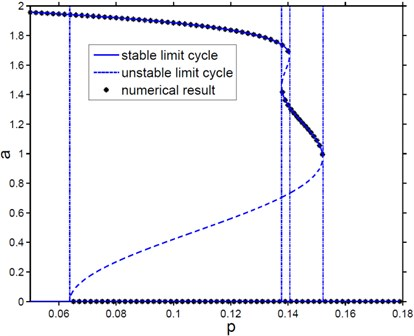Fig. 2Phase diagrams of the deterministic system (for 3 different values of p)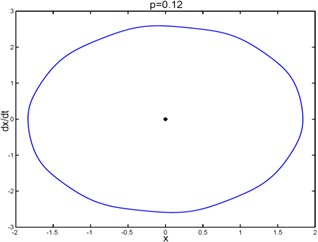a) Equilibrium and large limit cycle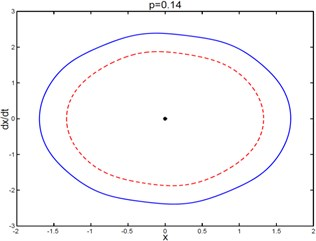b) Tri-stable state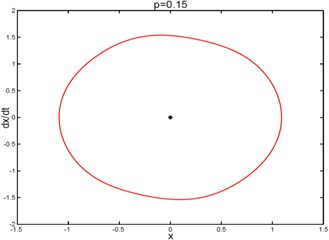c) Equilibrium and small limit cycle

Substituting Eq. (17) into Eq. (15), we can obtain:

18
$\left\{\begin{array}{l}da={F}_{11}\left(a,\theta \right)dt+{G}_{11}\left(a,\theta \right)\xi \left(t\right),\\ d\theta ={F}_{21}\left(a,\theta \right)dt+{G}_{21}\left(a,\theta \right)\xi \left(t\right),\end{array}\right\$

in which:

19
$\left\{\begin{array}{l}{F}_{11}=a\mathrm{s}\mathrm{i}{\mathrm{n}}^{2}\mathrm{\Phi }\left(-\epsilon +{\alpha }_{1}{a}^{2}\mathrm{c}\mathrm{o}{\mathrm{s}}^{2}\mathrm{\Phi }-{\alpha }_{2}{a}^{4}\mathrm{c}\mathrm{o}{\mathrm{s}}^{4}\text{Φ}+{\alpha }_{3}{a}^{6}\mathrm{c}\mathrm{o}{\mathrm{s}}^{6}\mathrm{\Phi }-{\alpha }_{4}{a}^{8}\mathrm{c}\mathrm{o}{\mathrm{s}}^{8}\mathrm{\Phi }\\ \mathrm{}\mathrm{}\mathrm{}\mathrm{}\mathrm{}-{w}^{p-1}\mathrm{s}\mathrm{i}\mathrm{n}\left(\frac{p\pi }{2}\right)\right),\\ {F}_{21}=\mathrm{s}\mathrm{i}\mathrm{n}\mathrm{\Phi }\mathrm{c}\mathrm{o}\mathrm{s}\mathrm{\Phi }\left(-\epsilon +{\alpha }_{1}{a}^{2}\mathrm{c}\mathrm{o}{\mathrm{s}}^{2}\mathrm{\Phi }-{\alpha }_{2}{a}^{4}\mathrm{c}\mathrm{o}{\mathrm{s}}^{4}\mathrm{\Phi }+{\alpha }_{3}{a}^{6}\mathrm{c}\mathrm{o}{\mathrm{s}}^{6}\mathrm{\Phi }-{\alpha }_{4}{a}^{8}\mathrm{c}\mathrm{o}{\mathrm{s}}^{8}\mathrm{\Phi }\mathrm{}\\ \mathrm{}\mathrm{}\mathrm{}\mathrm{}\mathrm{}-{w}^{p-1}\mathrm{s}\mathrm{i}\mathrm{n}\left(\frac{p\pi }{2}\right)\right),\\ {G}_{11}=-\frac{\mathrm{s}\mathrm{i}\mathrm{n}\mathrm{\Phi }}{{w}_{0}},\\ {G}_{21}=-\frac{\mathrm{c}\mathrm{o}\mathrm{s}\mathrm{\Phi }}{a{w}_{0}}.\end{array}\right\$

Eq. (18) can be regarded as a Stratonovich stochastic differential equation. By adding the corresponding Wong-Zakai correction term, we transform it into the following Itô stochastic differential equation:

20
$\left\{\begin{array}{l}da=\left[{F}_{11}\left(a,\theta \right)+{F}_{12}\left(a,\theta \right)\right]dt+{G}_{11}\left(a,\theta \right)dB\left(t\right),\\ d\theta =\left[{F}_{21}\left(a,\theta \right)+{F}_{22}\left(a,\theta \right)\right]dt+{G}_{21}\left(a,\theta \right)dB\left(t\right),\end{array}\right\$

where $B\left(t\right)$ is a standard Wiener process and:

21
$\left\{\begin{array}{l}{F}_{12}\left(a,\theta \right)=D\frac{\partial {G}_{11}}{\partial a}{G}_{11}+D\frac{\partial {G}_{11}}{\partial \theta }{G}_{21},\\ {F}_{22}\left(a,\theta \right)=D\frac{\partial {G}_{21}}{\partial a}{G}_{11}+D\frac{\partial {G}_{21}}{\partial \theta }{G}_{21}.\end{array}\right\$

By the stochastic averaging method , averaging Eq. (20) regarding $\mathrm{\Phi }$, the following averaged Itô equation can be obtained as follows:

22
$\left\{\begin{array}{l}da={m}_{1}\left(a\right)dt+{\sigma }_{1}\left(a\right)dB\left(t\right),\\ d\theta ={m}_{2}\left(a\right)dt+{\sigma }_{2}\left(a\right)dB\left(t\right),\end{array}\right\$

where:

23
$\left\{\begin{array}{l}{m}_{1}\left(a\right)={⟨{F}_{11}+D\frac{\partial {G}_{11}}{\partial a}{G}_{11}+D\frac{\partial {G}_{11}}{\partial \theta }{G}_{21}⟩}_{\mathrm{\Phi }},\\ {{\sigma }_{1}}^{2}\left(a\right)={⟨2D{{G}^{2}}_{11}⟩}_{\mathrm{\Phi }},\\ {m}_{2}\left(a\right)={⟨{F}_{21}+D\frac{\partial {G}_{21}}{\partial a}{G}_{11}+D\frac{\partial {G}_{21}}{\partial \theta }{G}_{21}⟩}_{\mathrm{\Phi }},\\ {{\sigma }_{2}}^{2}\left(a\right)={⟨2D{{G}^{2}}_{21}⟩}_{\mathrm{\Phi }}.\end{array}\right\$

Substituting Eq. (19) into Eq. (23), we obtain the explicit expression for the averaged drift and diffusion coefficients as follows:

24
$\left\{\begin{array}{l}{m}_{1}\left(a\right)=-\frac{1}{2}\left({w}^{p-1}\mathrm{s}\mathrm{i}\mathrm{n}\left(\frac{p\pi }{2}\right)+\epsilon \right)a+\frac{1}{8}{\alpha }_{1}{a}^{3}-\frac{1}{16}{\alpha }_{2}{a}^{5}+\frac{5}{128}{\alpha }_{3}{a}^{7}\\ \mathrm{}\mathrm{}\mathrm{}\mathrm{}\mathrm{}-\frac{7}{256}{\alpha }_{4}{a}^{9}+\frac{D}{2a{w}_{0}^{2}},\\ {{\sigma }_{1}}^{2}\left(a\right)=\frac{D}{{{w}^{2}}_{0}},\\ {m}_{2}\left(a\right)=0,\\ {{\sigma }_{2}}^{2}\left(a\right)=\frac{D}{{a}^{2}{{w}^{2}}_{0}}.\end{array}\right\$

Eqs. (22)-(24) show that the averaged Itô equation for $a\left(t\right)$ is independent of $\theta \left(t\right)$, the random process $a\left(t\right)$ is a one-dimensional diffusion process. The corresponding FPK equation associated with $a\left(t\right)$ can be wrote as:

25
$\frac{\partial p}{\partial t}=-\frac{\partial }{\partial a}\left[{m}_{1}\left(a\right)p\right]+\frac{1}{2}\frac{{\partial }^{2}}{\partial {a}^{2}}\left[{{\sigma }_{1}}^{2}\left(a\right)p\right].$

The boundary conditions are:

26
$\left\{\begin{array}{l}p=c,\mathrm{}\mathrm{}\mathrm{}\mathrm{}c\in \left(-\mathrm{\infty },+\mathrm{\infty }\right),a=0,\mathrm{}\\ p\to 0,\mathrm{}\mathrm{}\mathrm{}\mathrm{}\frac{\partial p}{\partial a}\to 0,a\to \mathrm{\infty }.\end{array}\right\$

Thus, based on these boundary conditions Eq. (26), we can obtain the stationary PDF of amplitude:

27
$p\left(a\right)=\frac{C}{{{\sigma }_{1}}^{2}\left(a\right)}\mathrm{e}\mathrm{x}\mathrm{p}\left[{\int }_{0}^{a}\frac{2{m}_{1}\left(u\right)}{{{\sigma }_{1}}^{2}\left(u\right)}du\right],$

where $C$ is a normalized constant, which satisfies:

28
$C={\left[{\int }_{0}^{\mathrm{\infty }}\left(\frac{1}{{{\sigma }_{1}}^{2}\left(a\right)}\mathrm{e}\mathrm{x}\mathrm{p}\left[{\int }_{0}^{a}\frac{2{m}_{1}\left(u\right)}{{{\sigma }_{1}}^{2}\left(u\right)}du\right]\right)da\right]}^{-1}.$

Substituting Eq. (24) into Eq. (27), we obtain the explicit expression for the stationary PDF of amplitude $a$:

29
$p\left(a\right)=\frac{Ca{{w}^{2}}_{0}}{D}\mathrm{e}\mathrm{x}\mathrm{p}\left[-\frac{{a}^{2}{{w}^{2}}_{0}\mathrm{\Delta }}{7680D}\right],$

in which:

30
$\mathrm{\Delta }=3840\epsilon +3840{w}^{p-1}\mathrm{s}\mathrm{i}\mathrm{n}\left(\frac{p\pi }{2}\right)-480{\alpha }_{1}{a}^{2}+160{\alpha }_{2}{a}^{4}-75{\alpha }_{3}{a}^{6}+42{\alpha }_{4}{a}^{8}.$

## 4. Stochastic P-bifurcation

Stochastic P-bifurcation refers to the changes in the number of peaks in the PDF curve. To obtain the critical parametric conditions for stochastic P-bifurcation, we analyze the influence of parameters on stochastic P-bifurcation of the system using singularity theory.

For the sake of convenience, we can write $p\left(a\right)$ as follows:

31
$p\left(a\right)=CR\left(a,\mathrm{}D,\mathrm{}\epsilon ,\mathrm{}w,\mathrm{}p,\mathrm{}{\alpha }_{1},\mathrm{}{\alpha }_{2},{\alpha }_{3},{\alpha }_{4}\right)\mathrm{e}\mathrm{x}\mathrm{p}\left[Q\left(a,\mathrm{}D,\mathrm{}\epsilon ,\mathrm{}w,\mathrm{}p,\mathrm{}{\alpha }_{1},\mathrm{}{\alpha }_{2},{\alpha }_{3},{\alpha }_{4}\right)\right],$

in which:

32
$\left\{\begin{array}{l}R\left(a,\mathrm{}D,\mathrm{}\epsilon ,\mathrm{}w,\mathrm{}p,\mathrm{}{\alpha }_{1},\mathrm{}{\alpha }_{2},{\alpha }_{3},{\alpha }_{4}\right)=\frac{Ca{{w}^{2}}_{0}}{D},\\ Q\left(a,\mathrm{}D,\mathrm{}\epsilon ,\mathrm{}w,\mathrm{}p,\mathrm{}{\alpha }_{1},\mathrm{}{\alpha }_{2},{\alpha }_{3},{\alpha }_{4}\right)=-\frac{{a}^{2}{{w}^{2}}_{0}}{7680D}\left(3840\epsilon +3840{w}^{p-1}\mathrm{s}\mathrm{i}\mathrm{n}\left(\frac{p\pi }{2}\right)\\ \mathrm{}\mathrm{}\mathrm{}\mathrm{}\mathrm{}\mathrm{}-480{\alpha }_{1}{a}^{2}+160{\alpha }_{2}{a}^{4}-75{\alpha }_{3}{a}^{6}+42{\alpha }_{4}{a}^{8}\right).\end{array}\right\$

According to singularity theory , the stationary PDF needs to satisfy the following two conditions:

33
$\frac{\partial p\left(a\right)}{\partial a}=0,\mathrm{}\mathrm{}\mathrm{}\frac{{\partial }^{2}p\left(a\right)}{\partial {a}^{2}}=0.$

Substituting Eq. (31) into Eq. (33), we can obtain the following condition [20, 38]:

34
$H=\left\{{R}^{\mathrm{\text{'}}}+R{Q}^{\mathrm{\text{'}}}=0,\mathrm{}\mathrm{}\mathrm{}R\mathrm{\text{'}}\mathrm{\text{'}}+2R\mathrm{\text{'}}Q\mathrm{\text{'}}+RQ\mathrm{\text{'}}\mathrm{\text{'}}+R{Q\mathrm{\text{'}}}^{2}=0\right\},$

where $H$ represents the condition for the changes of the number of peaks in the PDF curve.

Substituting Eq. (32) into Eq. (34), we can get the critical parametric conditions for stochastic P-bifurcation of the system with respect to fractional order$p$and noise intensity $D$ as follows:

35
$D=\frac{1}{4}{\alpha }_{1}{{w}^{2}}_{0}{a}^{4}-\frac{1}{4}{\alpha }_{2}{{w}^{2}}_{0}{a}^{6}+\frac{15}{64}{\alpha }_{3}{{w}^{2}}_{0}{a}^{8}-\frac{7}{32}{\alpha }_{4}{{w}^{2}}_{0}{a}^{10},$

where amplitude $a$ satisfies:

36
$128\epsilon -64{\alpha }_{1}{a}^{2}+48{\alpha }_{2}{a}^{4}-40{\alpha }_{3}{a}^{6}+35{\alpha }_{4}{a}^{8}+128{w}^{p-1}\mathrm{s}\mathrm{i}\mathrm{n}\left(\frac{p\pi }{2}\right)=0,$

with parameters $\epsilon =$ –0.1, ${\alpha }_{1}=$ 1.51, ${\alpha }_{2}=$ 2.85, ${\alpha }_{3}=$ 1.693, ${\alpha }_{4}=$ 0.312, $w=$ 1, according to Eqs. (35), (36), we obtain the transition set for stochastic P-bifurcation of the system with unfolding parameters $p$ and $D$ shown in Fig. 3.

Fig. 3Transition set curves of system Eq. (3)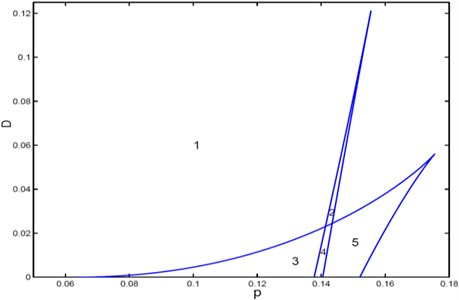According to singularity theory, the topological structures of the stationary PDF curves of different points ($p$, $D$) in the same region are qualitatively the same. By taking a point ($p$, $D$) in each region, we obtain all of the varieties of the stationary PDF curves that are qualitatively different. The unfolding parameter $p$-$D$ plane is divided into five sub-regions by the transition set curve; for convenience, each region in Fig. 3 is marked with a number.

We analyze the stationary PDF curves for a point ($p,D$) from each of the five sub-regions of Fig. 3, and show the corresponding results in Fig. 4.

Fig. 4Stationary PDF curves for the amplitude of system (3)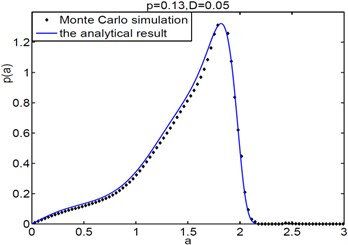a) Parameter ($p$, $D$) in region 1 of Fig. 3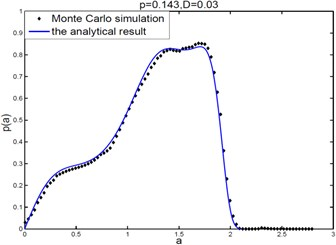b) Parameter ($p$, $D$) in region 2 of Fig. 3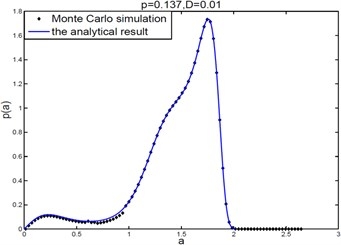c) Parameter ($p$, $D$) in region 3 of Fig. 3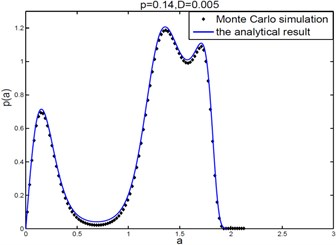d) Parameter ($p$, $D$) in region 4 of Fig. 3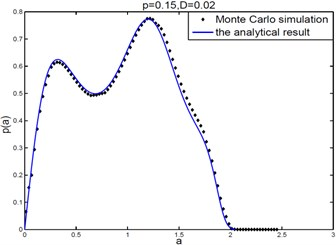e) Parameter ($p$, $D$) in region 5 of Fig. 3

As can be seen from Fig. 3, the parameter region where the PDF curve appears multimodal is surrounded by two approximately triangular regions. In particular, the region 4 where the two triangular regions are coincident forms a trimodal region of the stationary PDF curve for the system. When the parameter ($p$, $D$) is taken in the region 1, the PDF curve has a distinct peak far away from the origin, as shown in Fig. 4(a); in region 2, the PDF curve has two distinct peaks farther away from the origin, there are both small and large limit cycles in the system, as shown in Fig. 4(b); in region 3, the PDF curve still has a distinct peak farther away from the origin, but the probability is obviously not zero near the origin, there are both the equilibrium and large limit cycle in the system simultaneously, as shown in Fig. 4(c); in region 4, the PDF curve has three peaks, it shows that the equilibrium coexists with the small and large limit cycles in the system which is tri-stable, as shown in Fig. 4(d); in the region 5, the amplitude corresponding to the peak deviating from the origin of the PDF curve is smaller than the corresponding amplitude in Fig. 4(c), there are both the equilibrium and small limit cycle in the system simultaneously, as shown in Fig. 4(e).

The results show that the stationary PDF curves of the system amplitudes in any two adjacent regions in Fig. 3 are qualitatively different. Regardless of the exact values of the unfolding parameters, if they cross any line in the figure, the system will demonstrate stochastic P-bifurcation behavior. Therefore, the transition set curves are just the critical parametric conditions for the stochastic P-bifurcation of the system. The analytic results shown in Fig. 4 are in good agreement with the numerical results obtained by Monte Carlo simulation, further verifying the theoretical analysis and showing that it is feasible to use the methods in this paper to analyze the stochastic P-bifurcation behavior of fractional order system.

## 5. Conclusions

In this paper, we studied the tri-stable stochastic P-bifurcation of a generalized Van der Pol system with fractional derivative under Gaussian white noise excitation. According to the minimal mean square error principle, we transformed the original system into an equivalent integer-order system and obtained the stationary PDF of the system amplitude using stochastic averaging. In addition, we obtained the critical parametric conditions for stochastic P-bifurcation of the system using singularity theory, based on this, the system response can be maintained at the small amplitude near the equilibrium by selecting the appropriate unfolding parameters, providing theoretical guidance for system design in practical engineering and avoiding the instability and damage caused by the large amplitude vibration or nonlinear jump phenomenon of the system. The numerical results by Monte Carlo simulation of the original system also verify the theoretical results obtained in this paper. We conclude that the fractional order $p$ and noise intensity $D$ can each cause the stochastic P-bifurcation behavior of the system, and the number of peaks in the stationary PDF curve of system amplitude can change from one to three by selecting the appropriate unfolding parameters ($p$, $D$), it also shows that the method used in this paper is feasible to analyze the stochastic P-bifurcation behaviors of the nonlinear oscillator with fractional derivative.

#### Cited by

Fractional stochastic vibration system under recycling noise

02 August 2018
Accepted
09 January 2019
Published
15 May 2019
SUBJECTS
Fractional dynamics and applications
Keywords
Gaussian white noise
fractional derivative
stochastic P-bifurcation
transition set
Monte Carlo simulation
Acknowledgements

This work was supported by the National 973 Project of China (Grant No. 2014CB046805) and Natural Science Foundation of China (Grant No. 11372211).